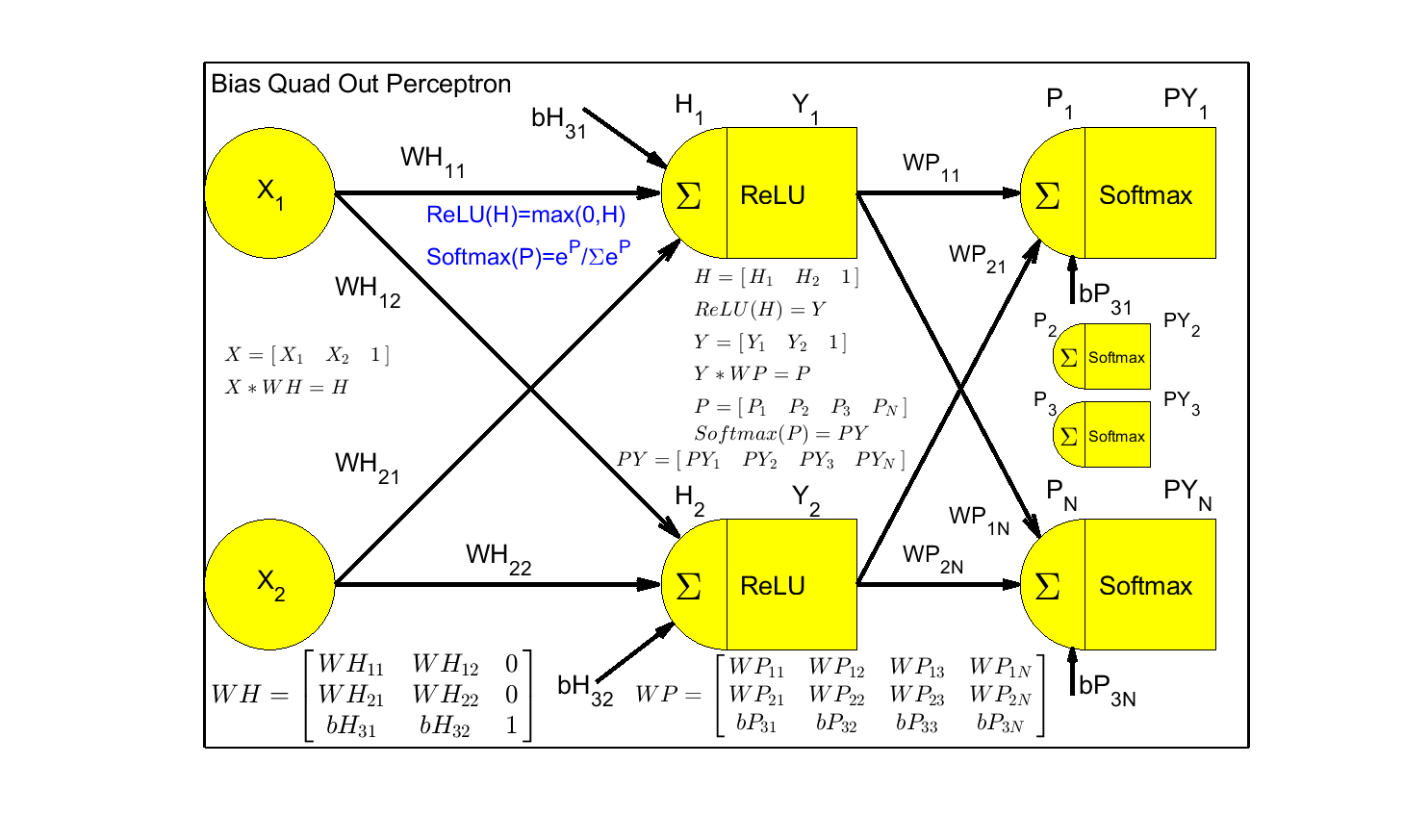# Problem 58907. Neural Net: Calculate Bias Quad Output Perceptron (Counter, Mux, Subtraction)

This challenge is to calculate the Neural Net Bias Quad Output Perceptron vector, PY, and Pclass, given X, WH, and WP using ReLU on the hidden layer and Softmax on the output layer. Test Cases will be all two input conditions for functions Counter, 2 bit Mux, and Subtraction 3-X_value. With four output classes ReLU performed better than Sigmoid in back propagation in ability to solve from random and prediction deltas between classes.
X wll be a 4x3 matrix creating a Quad output PY 4x4 matrix. PY(:,N) is Expectation of class N for each case. PY values shall all be <0.25 or >.75 The Pclass must match the Logical Function expectation where Pclass is the column of PY with max value per row, col1=Class1, col2=Class2, col3=Class3, col2=Class4.The function template includes the code to create this figure using Matlab Latex

### Solution Stats

100.0% Correct | 0.0% Incorrect
Last Solution submitted on Sep 26, 2023

### Community Treasure Hunt

Find the treasures in MATLAB Central and discover how the community can help you!

Start Hunting!